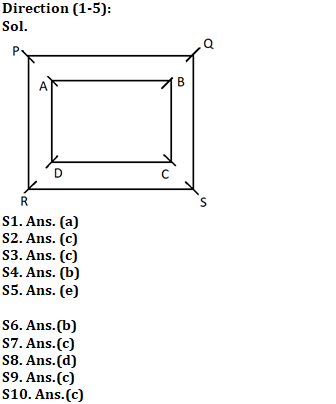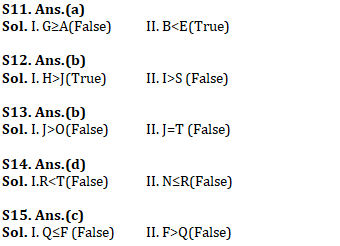Latest Banking jobs   »

# Reasoning Ability Quiz For IBPS RRB PO Prelims 2022- 18th June

Directions (1-5): Study the following information carefully and answer the questions given below:
Eight persons i.e., P, Q, R, S, A, B, C and D sit at the corner of two square tables which one inside the other. Four persons sit at the corner of outer table and are facing towards the center of table while rest sitat the corner of inner table and are facing away from the center of table.
P faces the one who sits to the immediate right of D. S does not sit next to P but sits on the same table on which P sits. B sits to the immediate left of C. B does not face P. One person sits between R and Q. Q does not face D. R faces towards the center of table.

Q1. Four of the following five are alike in a certain way and hence they form a group. Which one of the following does not belong to that group?
(a) P-S
(b) P-A
(c) R-D
(d) S-C
(e) Q-B

Q2. Who among the following faces C?
(a) A
(b) B
(c) S
(d) P
(e) None of these

Q3. The number of persons sits between P and R when counted from the left of P, is same as the number of persons sits between B and ___ when counted from the right of ___?
(a) Q
(b) A
(c) C
(d) S
(e) None of these

Q4. How many persons sits between A and C?
(a) Two
(b) One
(c) Three
(d) Four
(e) None of these

Q5. Who among the following sits to the immediate left of A?
(a) P
(b) Q
(c) R
(d) B
(e) None of these

Directions (6–10): Study the following arrangement carefully and answer the questions given below:

7 D 5 # A B 1 % K \$ 4 E J F € &2 H I @ L 6 Q U © 9 M T 8 W

Q6. How many such symbols are there in the above arrangement each of which is immediately preceded by a vowel and also immediately followed by a number?
(a) None
(b) One
(c) Two
(d) Three
(e) Four

Q7. Four of the following five are alike in a certain way on the basis of their positions in the above arrangement and so form a group. Which is the one that does not belong to the group?
(a) K41
(b) &HF
(c) #B5
(e) LQI

Q8. Which of the following pairs of elements has the second element immediately followed by the first element?
(a) 5#
(b) MT
(c) \$4
(d) Q6
(e) @L

Q9. How many such numbers are there in the above arrangement, each of which is immediately followed by a symbol and also immediately preceded by a consonant?
(a) None
(b) One
(c) Two
(d) Three
(e) More than three

Q10. If all the symbols are removed, then which of the following element is 6th to the right of the element which is 10th from the left end?
(a) Q
(b) H
(c) 6
(d) L
(e) None of these

Directions (11-15): In these questions, a relationship between different elements is shown in the statements. The statements are followed by two conclusions. Give answer

Q11. Statement: A>B; G<D≤E; G≥F>B
Conclusion: I. G≥A
II. B<E

(a) if only conclusion II is true.
(b) if only conclusion I is true.
(c) if neither conclusion I nor II is true.
(d) if either conclusion I or II is true.
(e) if both conclusions I and II are true.

Q12. Statement: A>T≥J; A≤S=H; I>T
Conclusion: I. H>J
II. I>S

(a) if both conclusion I and II are true.
(b) if only conclusion I is true.
(c) if neither conclusion I nor II is true.
(d) if either conclusion I or II is true.
(e) if only conclusion II is true.

Q13. Statement: J≥K>L<M≥N; K≥O=T
Conclusion: I. J>O
II. J=T

(a) if only conclusion II is true.
(b) if either conclusion I or II is true.
(c) if neither conclusion I nor II is true.
(d) if only conclusion I is true.
(e) if both conclusions I and II are true.

Q14. Statement: A>T=N; A>S>R; A<M
Conclusion: I.R<T
II. N≤R

(a) if only conclusion II is true.
(b) if only conclusion I is true.
(c) if neither conclusion I nor II is true.
(d) if either conclusion I or II is true.
(e) if both conclusions I and II are true.

Q15. Statement: A<B≤C; F<M≤C; C>Q
Conclusion: I. Q≤F
II. F>Q

(a) if only conclusion II is true.
(b) if either conclusion I or II is true.
(c) if neither conclusion I nor II is true.
(d) if only conclusion I is true.
(e) if both conclusions I and II are true.

Solutions#### Congratulations!